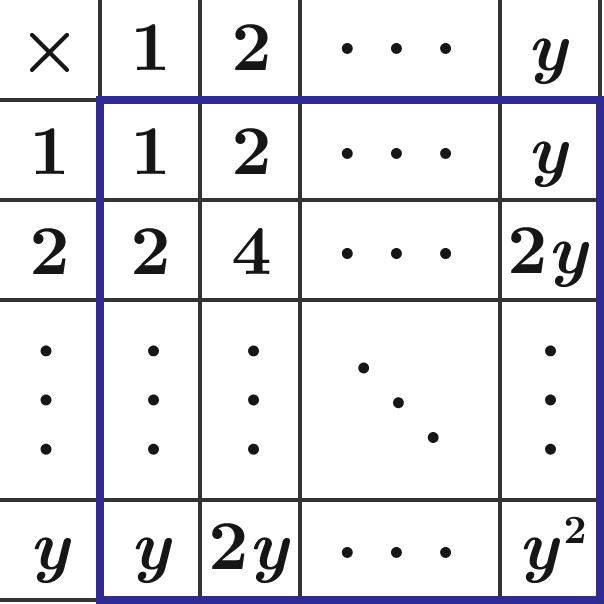Algebra Level 1

Below is a multiplication table up to $y \times y.$ Find $y$ such that the sum of all the products in the blue square equals 90000.×